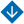Using Piecewise Functions to Normalize Input Variables of Fuzzy Inference Systems
•About Advisory & Editorial Boards Aims & Scope Publication Ethics Review Process Archive Contact Information

#Using Piecewise Functions to Normalize Input Variables of Fuzzy Inference Systems

Sorokin, A.A. Using Piecewise Functions to Normalize Input Variables of Fuzzy Inference Systems

Abstract. This paper proposes a method for normalizing the input variables of fuzzy inference systems (FISs), which are used in assessing integrally the state of a complex object. The method involves piecewise functions: the variable’s range is divided into several intervals (the length of each interval depends the variable’s specifics), and a particular function is assigned to each interval. This function shows the patterns of the variable’s variations on the normalized scale relative to its variations on the absolute scale. The set of these functions for the entire range of the variable forms the normalization operator. When implementing the normalization operator, the functions are selected so that after transformation, all input variables positively correlate with the output variable. This approach simplifies the construction of FISs: the same terms of the input variables have the same semantic meaning after transformation. According to the simulation results, FISs with the proposed normalization method are adequate to similar FISs without the normalization of the input variables. The proposed normalization method allows reducing the number of rules in the FIS knowledge base if the input variables have an optimum of their influence on the value of the output variable.

Keywords: fuzzy inference system, normalization, input variable, knowledge base, rule, integral assessment, information processing.

PDF (English)